# Electronics and Communication Engineering - Exam Questions Papers

1.

A ramp input applied to a unity feedback system results in 5% steady state error. The type number and zero frequency gain of the system are respectively.

 A. 1 and 20 B. 0 and 20 C. 0 and 1/20 D. 1 and 1/20

Explanation:

ess = 5% ⇒ .05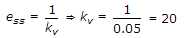.

2.

A fair coil is tossed 10 times. What is the probability that ONLY the first two tosses will yield heads?

 A.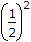B.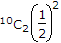C.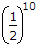D.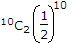Explanation:

Use ncr, (p)r (q)n-r [for any two losses yield head].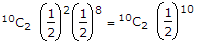But in present case it is required case it is only for first two tosses. Thus in this case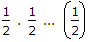10 times.

3.

The equation sin (z) = 10 has

 A. no real or complex solution B. exactly two distinct complex solutions C. a unique solution D. an infinite number of complex solutions

Explanation:

Since value lies between - 1 and + 1. Therefore no real or complex solution exists.

4.

Given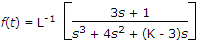If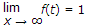, then the value of K is

 A. 1 B. 2 C. 3 D. 4

Explanation: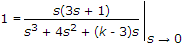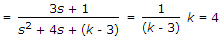.

5.

Consider the Schmitt trigger circuit shown below. A triangular wave which goes from - 12 V to 12 V is applied to the inverting input of the op-amp. Assume that the output of the op-amp moves from +15 V to -15 V. The voltage at the non-inverting switches between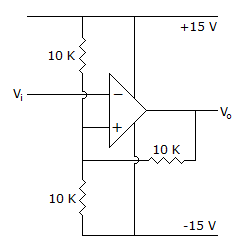A. -12 V and +12 V B. -7.5 V and +7.5 V C. -5 V and +5 V D. 0 V and 5 V

Explanation:

V0 is switching between + 15 to - 150

Thus voltage at non-inverting input switches between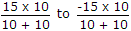, 7.5 to - 7.5 .